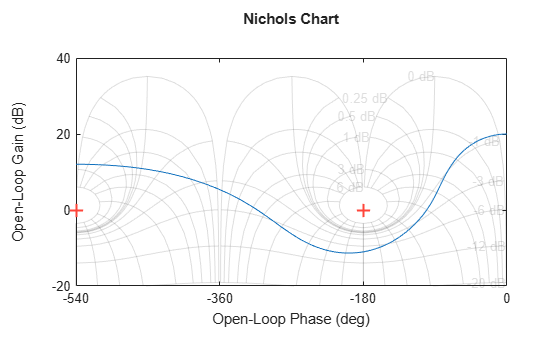ngrid

Superimpose Nichols chart on Nichols plot

Description

example

ngrid superimposes Nichols chart grid lines over the Nichols frequency response of a SISO LTI system. The range of the Nichols grid lines is set to encompass the entire Nichols frequency response.

The chart relates the complex number H/(1 + H) to H, where H is any complex number. For SISO systems, when H is a point on the open-loop frequency response, then the corresponding value of the closed-loop frequency response assuming unit negative feedback is

$\frac{H}{1+H}$

If the current axis is empty, ngrid generates a new Nichols chart grid in the region –40 dB to 40 dB in magnitude and –360 degrees to 0 degrees in phase. ngrid returns a warning if the current axis does not contain a SISO Nichols frequency response.

ngrid('new') clears the current axes first and sets hold on.

ngrid(AX,___) plots the grid on the Axes or UIAxes object in the current figure with the handle AX. Use this syntax when creating apps with ngrid in the App Designer.

Examples

collapse all

Plot the Nichols response with Nichols grid lines for the following system:

$H\left(s\right)=\frac{-4{s}^{4}+48{s}^{3}-18{s}^{2}+250s+600}{{s}^{4}+30{s}^{3}+282{s}^{2}+525s+60}.$

H = tf([-4 48 -18 250 600],[1 30 282 525 60]);
nichols(H)
ngridThe context menu for Nichols charts includes the Tight option under Zoom. You can use this option to clip unbounded branches of the Nichols chart.

Input Arguments

collapse all

Object handle, specified as an Axes or UIAxes object. Use AX to create apps with ngrid in the App Designer.